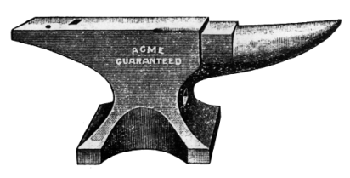# Hesiod’s AnvilHow far off is heaven? In the Theogony Hesiod gives us a clue:

For a brazen anvil falling down from heaven nine nights and days would reach the earth upon the tenth; and again, a brazen anvil falling from earth nine nights and days would reach Tartarus upon the tenth.

How far can an anvil fall in nine days? Galileo, who taught that “the distances measured by the falling body increase according to the squares of the time,” would have determined that the anvil starts 2.96 × 109 km from earth, a distance greater than that between the sun and Uranus.

But Galileo’s calculation assumes that gravitational force is independent of the object’s distance from the earth. If we assume instead that it varies inversely with the square of the distance between mass centers (and if we ignore all masses except those of the earth and the anvil, and assume that the anvil falls in a straight line), King College mathematician Andrew Simoson calculates that Galileo’s anvil wouldn’t reach us forInstead, under this new assumption, to reach us in nine days an anvil would start 5.81 × 105 km away — about one and a half times the distance between the earth and the moon.

(Andrew J. Simoson, Hesiod’s Anvil, 2007.)